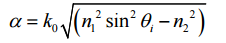# Attenuation Factor of an Optical Fibre Calculator

This CalcTown calculator calculates the attenuation factor of an optical fibre.

degree
m-1

#### Result

dB/mwhere

θi is the angle the ray makes with the fiber axis, before entering the fiber,

k0 is the wavenumber of the EM wave in vacuum,

n1 is the refractive index along the central axis of the fiber, and

n2 is the refractive index of the cladding.